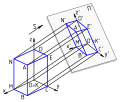# Cross section (geometry)

Cross section (geometry)
Graphical projectionPart of a series on: Planar Parallel projection Orthographic projection Multiviews Plan, or floor plan Section Elevation Auxiliary Axonometric projection (i.e. pictorials) Isometric projection Dimetric projection Trimetric projection Oblique projection Cavalier projection Cabinet projection Perspective projection Linear perspective One-point perspective Two-point perspective Three-point perspective Zero-point perspective Curvilinear perspective Reverse perspective Views Bird's-eye view/Aerial view Detail view 3/4 perspective Cutaway drawing Exploded view drawing Fisheye Fixed 3D Panorama Top-down perspective Worm's-eye view Zoom v · d · e

In geometry, a cross-section is the intersection of a figure in 2-dimensional space with a line, or of a body in 3-dimensional space with a plane, etc. More plainly, when cutting an object into slices one gets many parallel cross-sections.

Cavalieri's principle states that solids with corresponding cross-sections of equal areas have equal volumes.

The cross-sectional area (A') of an object when viewed from a particular angle is the total area of the orthographic projection of the object from that angle. For example, a cylinder of height h and radius r has A' = πr2 when viewed along its central axis, and A' = 3πrh when viewed from an orthogonal direction. A sphere of radius r has A' = πr2 when viewed from any angle. More generically, A' can be calculated by evaluating the following surface integral:$A' = \iint \limits_\mathrm{top} d\mathbf{A} \cdot \mathbf{\hat{r}},$

where$\mathbf{\hat{r}}$ is a unit vector pointing along the viewing direction toward the viewer,$d\mathbf{A}$ is a surface element with outward-pointing normal, and the integral is taken only over the top-most surface, that part of the surface that is "visible" from the perspective of the viewer. For a convex body, each ray through the object from the viewer's perspective crosses just two surfaces. For such objects, the integral may be taken over the entire surface (A) by taking the absolute value of the integrand (so that the "top" and "bottom" of the object do not subtract away, as would be required by the Divergence Theorem applied to the constant vector field$\mathbf{\hat{r}}$) and dividing by two:$A' = \frac{1}{2} \iint \limits_A | d\mathbf{A} \cdot \mathbf{\hat{r}}|$